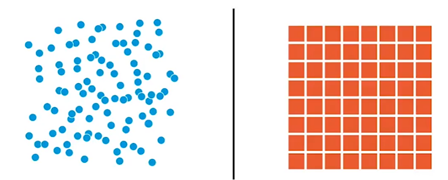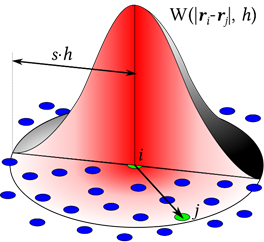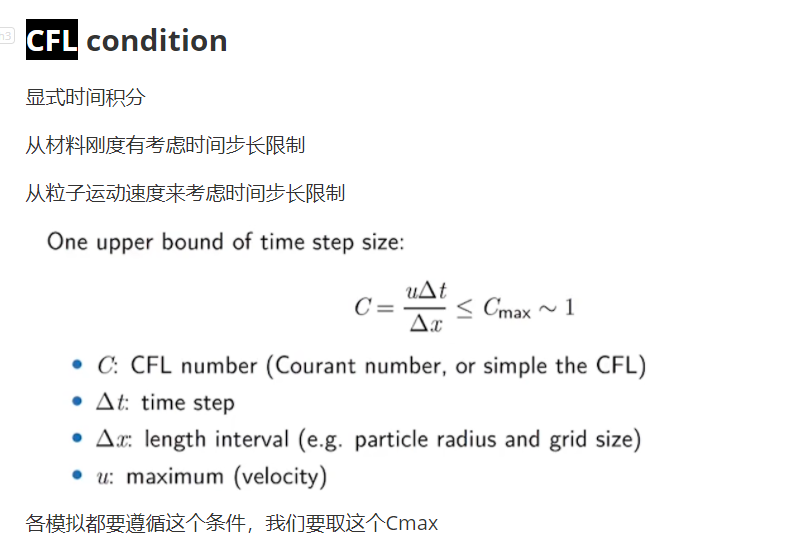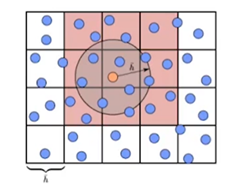# 物理模拟——PBF流体模拟（1）

## 介绍

PBF全称Position Based Fluid，意为基于位置的流体，是基于Position Based Dynamics实现的流体模拟，本文会讲解物理动画概念、流体模拟基础、SPH、PBD、PBF以及流体渲染。

## 基于物理的动画

### 流体动画

3d游戏中的流体动画一般的实现比较复杂，不同规模的流体有不同的做法，甚至不同的材料都会使用不同的模拟方法。

## 流体模拟基础

### 梯度

$\nabla f(x,y) =(\frac {\partial f}{\partial x},\frac {\partial f}{\partial y})$

3维的梯度见式：

$\nabla f(x,y,z) =(\frac {\partial f}{\partial x},\frac {\partial f}{\partial y},\frac {\partial f}{\partial z})$

### 散度

$\nabla \cdot \vec{u} = \nabla \cdot (u,v) = \frac{\partial u}{\partial x}+ \frac {\partial v}{\partial y}$

### 旋度

$\nabla \times \vec{u} = \nabla \times (u,v) = \frac{\partial v}{\partial x}- \frac {\partial u}{\partial y}$

### 拉普拉斯算子

$\nabla \cdot \nabla f =\frac{\partial^2 f}{\partial x^2}+ \frac {\partial^2 f}{\partial y^2}$

### 粘性

$\mu$ 动力粘性系数表示相同变形率是的粘性大小， $\upsilon$ 运动粘性系数表示的是相同加速度时的粘性大小，在Navier-Stokes方程中出现的即是运动粘性系数。

### 纳维-斯托克斯偏微分方程组

$\frac{\partial \vec{u}}{\partial {t}} + \vec{u}\cdot\nabla\vec{u}+\frac{1}{\rho}\nabla p = \vec{g}+\upsilon \nabla \cdot \nabla \vec{u}$

$\nabla \cdot \nabla \vec{u} = 0$

Navier-Stokes方程是一个二阶非线性偏微分方程，求解起来非常复杂，在模拟领域使用计算机对NS方程进行求解，通常将方程分解为几个部分，然后按顺序推进。

### 拉格朗日视角和欧拉视角## SPH

### 原理及公式

Smoothed-Particle Hydrodynamics光滑粒子流体动力学是一种用来模拟连续介质动力学的离散方法，他属于拉格朗日法，最初用于天体物理领域，近年来在计算机图形学领域热度逐渐提高，常用来模拟流体运动。由于其无网格的特性，SPH在模拟复杂边界、扭曲拉伸、湍流上具有天然的优势。

SPH的高层思想就是使用一堆随着模拟运动而更新位置的粒子去携带物理量，使用核函数来近似一个连续的场，某一个位置的物理量的值等于周围粒子的物理量的加权平均，核函数就是用来决定权值，距离该位置越近的权值越大。$A(x) = \sum _i A_i \frac{m_i}{\rho_i}W(||x-x_j||_2,h)$

$\nabla A_i =\rho_i \sum _j {(\frac{A_i}{\rho_i^2}+\frac{A_j}{\rho_j^2}) \nabla_{x_i} W(||x_i-x_j||_2,h))}$

$\frac{Dv}{Dt} = -\frac{1}{\rho}\nabla p +g$

$\rho(x) = \sum _i {m_i}W(||x-x_j||_2,h)$

$p = B((\frac{\rho}{\rho_0})^\gamma - 1)$

B：bulk modulus，$\rho_0$ 是一个理想密度，密度很大压强就很大，压强很高就会尝试推着周围的粒子离开自己。

### 过程

1、对每个粒子计算它所在位置的密度；

2、对每个粒子计算它的压强梯度，进而得到它的Dv/Dt（其实就相当于求一个力）；

3、Symplectic Euler Step半隐式欧拉方法推进模拟，先根据算出来的力更新速度，然后用更新过的速度来更新位移。### 加速

SPH算法在使用过程中一般最大瓶颈就在粒子的邻居搜索，因为粒子随模拟运动而运动，邻居在每次算法执行前都需要进行更新，要精确的找到一个粒子的周围粒子这个直接暴力的时间复杂度是O（n^2），为了降低这个瓶颈带来的影响加速SPH算法，一般会使用特殊的数据结构来存储粒子邻居，比如一个将位置信息通过哈希算法投射到一个数组中，一般的步骤是先将连续的粒子位置信息离散为几个大块，分成格子，然后做格子位置到粒子数组的映射，格子之间访问邻居非常简单只需要加上偏移量。这样一来一个粒子周围的粒子就等于他所在的格子的所有粒子加上周围一圈格子的所有粒子，每次算法结束步骤时进行维护，根据位置再对格子里的粒子做一个更新。SPH邻居搜索的加速方法是很多，比如使用CPU并行进行邻居搜索、使用CUDA等GPU计算来加速。

## 引用

https://yangwc.com/2019/05/01/fluidSimulation/

https://diglib.eg.org/bitstream/handle/10.2312/PE.PG.PG2012short.029-034/029-034.pdf

https://book.douban.com/subject/35287308/

2021.8.25

posted @ 2021-08-25 00:40  飞翔的子明  阅读(1033)  评论(1编辑  收藏  举报